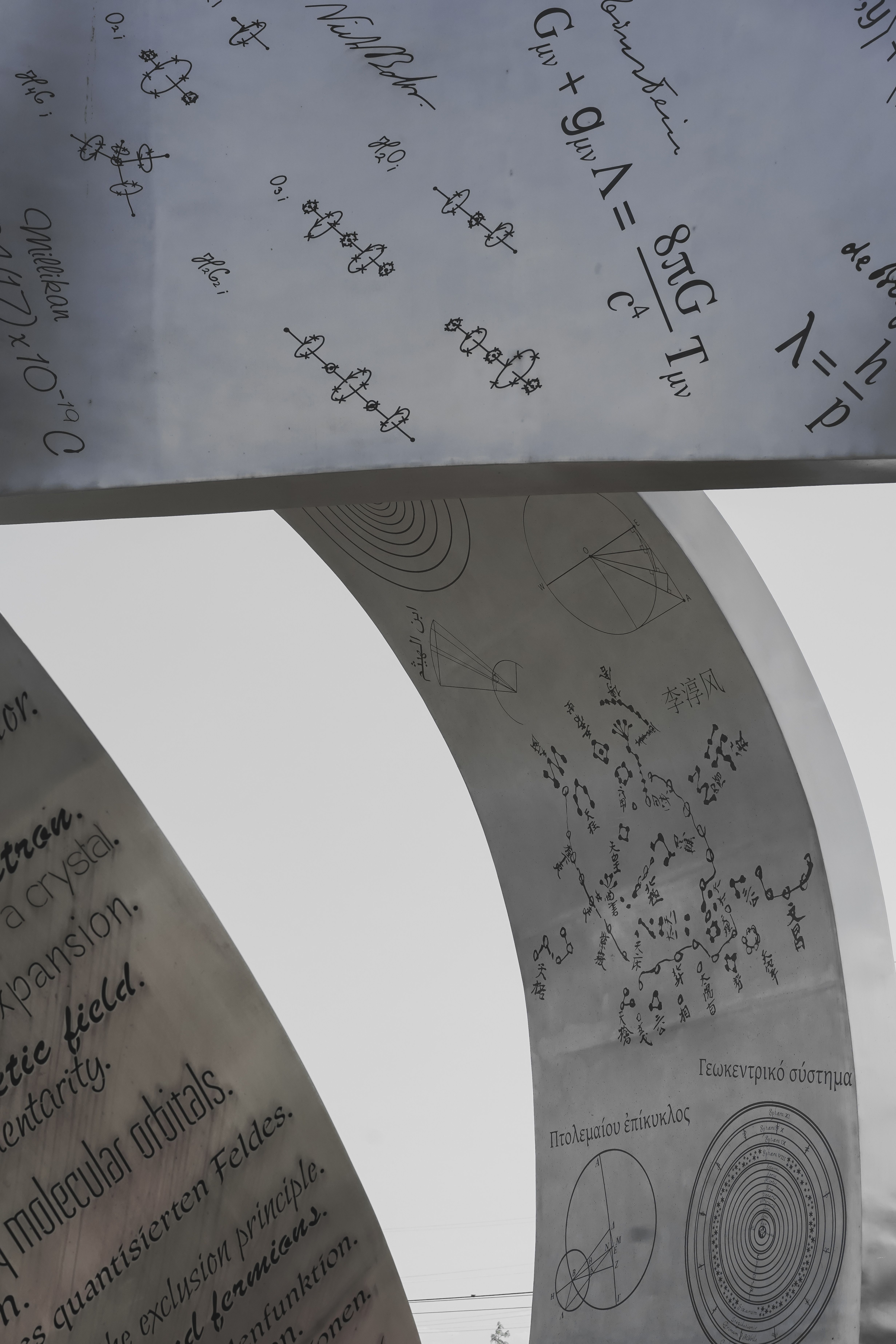# What level of math is physics?

What Level of Math is Physics? – College Tips and Tricks Blogger

# What Level of Math is Physics?Physics is a challenging subject that requires solid math skills. Math is the language of physics, and it helps you understand the concepts and solve problems. But, what level of math is physics? In this article, we’ll explore the math skills you need for physics and how you can improve them to succeed in this exciting field.

## FAQ

### What math is used in physics?

The math used in physics ranges from algebra to calculus and beyond. Some of the essential math skills you’ll need in physics include:

• Algebra: You need to have a good understanding of basic algebraic operations such as simplification of equations, solving equations, and manipulating expressions.
• Trigonometry: You need to know the basic trigonometric functions, such as sine, cosine, and tangent, and how they relate to right triangles.
• Calculus: Calculus will help you solve many physics equations. You’ll start with single-variable calculus, then progress to multivariable calculus. The latter is extremely relevant to physics because you’ll work with directional derivatives and similar concepts in three-dimensional space.

### Why is math important in physics?

Math is an essential tool for physics because it helps you describe and understand the fundamental laws of nature. The laws of physics are expressed in mathematical equations, and without a solid math foundation, you will have a hard time understanding and applying these laws. Math skills are also crucial for solving physics problems, which require a combination of conceptual understanding and mathematical reasoning.

### What are some tips for improving math skills for physics?

Improving your math skills takes time and effort, but it’s worth it if you want to succeed in physics. Here are some tips for improving your math skills:

• Practice regularly: Practice is the key to improving your math skills. Set aside some dedicated time each day or week to practice math problems.
• Master the basics: Make sure you have a solid foundation in algebra, trigonometry, and calculus basics. These fundamentals will help you understand more advanced concepts.
• Get help when needed: Don’t be afraid to ask for help if you’re struggling with math. You can seek help from tutors, teachers, or online resources.
• Apply math to physics problems: Start solving physics problems that require math skills. This will help you understand how math is used in physics and improve your problem-solving skills.

## Comparing Different Levels of Math for Physics

Math Level Description Examples
Algebra Basic algebraic operations such as equations, expressions, and simplification. Finding the value of x in an equation, simplifying expressions.
Trigonometry Study of triangles and the relationships between their sides and angles. Finding the angle or side length of a right triangle using trigonometric functions.
Calculus Study of rates of change and accumulation using limits, derivatives, integrals, and infinite series. Finding the derivative of a function, solving optimization problems using derivatives, finding the area under a curve using integrals.

## How Math is Used in PhysicsMath is used extensively in physics to describe the laws of nature and solve problems. The laws of physics are expressed in mathematical equations, and you need math skills to understand and apply these laws.

For example, Newton’s second law of motion, F = ma, relates the force applied to an object to its acceleration. This equation requires algebra and the knowledge of how to use vectors to represent force and acceleration. Another example is Ohm’s law, which states that the current through a conductor between two points is proportional to the voltage across the two points. This equation requires algebra and the concept of proportions.

When solving physics problems, you need to use math skills to manipulate and solve equations. For example, you may need to solve for an unknown variable such as velocity, acceleration, or force. This requires algebraic manipulation and the knowledge of basic trigonometric functions. In more advanced physics problems, you may need to use calculus and other advanced math skills to solve equations, such as finding the derivative or integral of a function.

## Ways to Improve Math Skills for PhysicsIf you’re struggling with math skills for physics, there are several ways you can improve:

• Practice regularly: Practice is the key to improving your math skills. Set aside some dedicated time each day or week to practice math problems.
• Master the basics: Make sure you have a solid foundation in algebra, trigonometry, and calculus basics. These fundamentals will help you understand more advanced concepts.
• Get help when needed: Don’t be afraid to ask for help if you’re struggling with math. You can seek help from tutors, teachers, or online resources.
• Apply math to physics problems: Start solving physics problems that require math skills. This will help you understand how math is used in physics and improve your problem-solving skills.
• Take good notes: Take good notes during class and review them regularly. This will help you reinforce your math skills and understand how the concepts relate to physics problems.
• Use online resources: There are many online resources available for improving math skills for physics, such as Khan Academy, Mathway, and Wolfram Alpha.